# Boolean algebra facts for kids

Kids Encyclopedia Facts

In mathematics, Boolean algebra is an algebra for binary digits (where 0 means false and 1 means true). It is equipped with three operators: conjunction (AND), disjunction (OR) and negation (NOT). It uses normal math symbols, but it does not work in the same way. It is named for George Boole, who invented it in the middle 19th century. Boolean algebra did not get much attention except from mathematicians until the 20th century when engineers began using it for logic gates.

## NOT gate

NOT
0 1
1 0

The NOT operator is written with a bar over numbers or letters like this: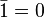$\bar{1} = 0$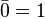$\bar{0} = 1$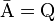$\bar{\mbox{A}} = \mbox{Q}$

It means that the output is not the input.

## AND gate

AND 0 1
0 0 0
1 0 1

The AND operator is written as$\cdot$ like this: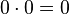$0 \cdot 0 = 0$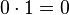$0 \cdot 1 = 0$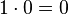$1 \cdot 0 = 0$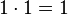$1 \cdot 1 = 1$

The output is true if and only if one and the other input is true.

## OR gate

OR 0 1
0 0 1
1 1 1

The OR operator is written as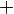$+$ like this: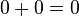$0 + 0 = 0$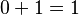$0 + 1 = 1$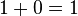$1 + 0 = 1$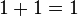$1 + 1 = 1$

If one or the other input is true, then the output to be true (and false otherwise).

## XOR gate

XOR 0 1
0 0 1
1 1 0

XOR basically means "exclusive or", meaning one input or the other must be true, but not both. It is also sometimes called NOR, which means the same thing.

The XOR operator is written as$-$ like this: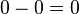$0 - 0 = 0$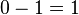$0 - 1 = 1$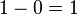$1 - 0 = 1$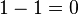$1 - 1 = 0$

In other words, the XOR operator returns true precisely when one or the other input is true—but not both.

## Identities

Different gates can be put together in different orders: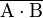$\overline{\mbox{A} \cdot \mbox{B}}$ is the same as an AND then a NOT. This is called a NAND gate.

It is not the same as a NOT then an AND: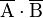$\overline{\mbox{A}} \cdot \overline{\mbox{B}}$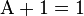$\mbox{A} + 1 = 1$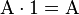$\mbox{A} \cdot 1 = \mbox{A}$

which is called XOR identity table

XOR 1 0 Any
1 TRUE 0 0
0 0 0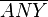$\overline{ANY}$
Any 0$\overline{ANY}$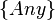$\{Any\}$

, if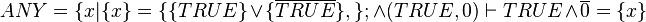$ANY=\{x|\{x\}=\{\{TRUE\}\or\{\overline{TRUE}\}, \};\and (TRUE, 0) \vdash TRUE \and \overline{0} = \{x\}$. or if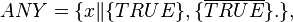$ANY=\{x \|\{TRUE\}, \{\overline{TRUE}\} .\},$=TRUE, TRUE.,

## De Morgan's laws

Augustus De Morgan discovered that it is possible to preserve the truth values of Boolean expressions by changing a$+$ sign to a$\cdot$ sign, while making or breaking a bar. That is: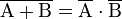$\overline{\mbox{A} + \mbox{B}} = \overline{\mbox {A}} \cdot \overline{\mbox{B}}$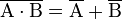$\overline{\mbox{A} \cdot \mbox{B}} = \overline{\mbox {A}} + \overline{\mbox{B}}$

These findings are commonly known as De Morgan's laws.

## Images for kidsBoolean algebra Facts for Kids. Kiddle Encyclopedia.